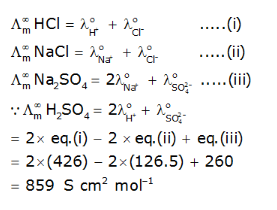# Calculate molar conductivity at infinite dilution of H2SO4

calculate molar conductivity at infinite dilution of H2SO4. given molar conductivity at infinite dilution of HCl = 426, molar conductivity at infinite dilution of NaCl = 126.5 and molar conductivity at infinite dilution of Na2SO4 =260 at 25-degree celcius. (units are scm2/mol)